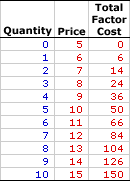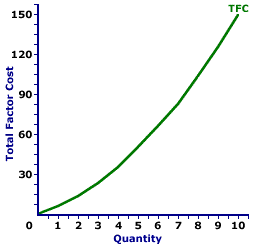Tuesday  October 26, 2021
 AmosWEB means Economics with a Touch of Whimsy!AAUP: The abbreviation for American Association of University Professors, which is an association of university and college faculty established in 1915 to protect academic freedom. The AAUP is the closest thing university faculty have to a labor union. While it does engage in some collective bargaining functions with specific universities, similar to traditional labor unions, its primary function is to ensure that faculty maintain intellectual or academic freedom from political of social pressures.TOTAL FACTOR COST, MONOPSONY:

The opportunity cost incurred by a monopsony when using a given factor of production to produce a good or service. This is the total cost associated with the use of a particular resource or factor of production--it is the total cost of the factor. For monopsony, the price paid increases with the quantity purchased and total factor cost increases at an increasing rate. Total factor cost is predominately used in the analysis of the factor market. Two derivative factor cost measures are average factor cost and marginal factor cost.
Total factor cost is the total opportunity cost incurred by a monopsony from the employment of a given resource. This measure of cost needs to be contrasted with a similar, better known term, total cost. Total cost is the cost of all factors of production, whereas total factor cost is the cost of using a specific factor. Total cost, as such, is the sum of the total factor cost of labor, the total factor cost of capital, and the total factor cost of all other inputs.

Total factor cost can be represented in a table or as a curve. For a firm with no market control hiring inputs under perfect competition, the total factor cost curve is a straight line that emerges from the origin. For firms with market control, including monopsony, oligopsony, or monopsonistic competition, the total factor cost curve increases at an increasing rate. The shape of the total factor cost curve thus indicates the degree of market control possessed by the factor buyer.

Whichever market structure is involved, total factor cost is calculated as the factor price times the quantity of the factor purchased, as illustrated by this equation:

 total factor cost = factor price x factor quantity
If the firm is hiring the factor in a perfectly competitive factor market, then the factor price is fixed or constant and total factor cost increases at a constant rate. If the firm is hiring the factor in an imperfectly competitive factor market, best illustrated by monopsony, then the factor price increases with larger factor quantities and the total factor cost increases at an increasing rate.

For a market structure like monopsony that has some degree of market control and is a price maker rather than a price taker, total factor cost increases at an increasing rate. Although total factor cost is also calculated as price times quantity, market control means monopsony faces a positively-sloped supply curve. As such, the price received is not fixed, but depends on the quantity of the input bought.

Total Factor Cost,
MonopsonyThe table to the right summarizes the total factor cost received by a hypothetical firm, OmniKing Island Resort. This firm is the only employer of labor on a small tropical island. As the only employer of labor on the Island, OmniKing is a monopsony with extensive market control, and it faces a positively-sloped supply curve. To employ more workers, OmniKing must pay a higher price.

Consider a few tidbits of information about total factor cost for a firm with market control, such as OmniKing Island Resort.

• First, total factor cost is zero if OmniKing hires no labor. This makes sense. If nothing is bought, no factor cost is incurred.

• Second, the price changes with the quantity of output bought. OmniKing can hire 1 worker for \$6. However, if it choses to hire 5 workers, then it must raise the price to \$10.

• Third, as OmniKing hires more labor it pays more total factor cost.

• Fourth and last, the extra factor cost incurred from hiring more labor is not constant nor is it equal to the price. For example, the fifth worker adds a extra \$14 to factor cost (the difference between \$50 and \$36) even though the price is \$10.
Total Factor Cost Curve,
MonopsonyThe total factor cost curve for the OmniKing Island Resort monopsony is displayed in the exhibit to the right. Key to this curve is that OmniKing is a monopsony buyer of workers and thus faces a positively-sloped supply curve. Larger quantities of input can be had only with higher prices.

The vertical axis measures total factor cost and the horizontal axis measures the quantity of input (workers). Although quantity on this particular graph stops at 10 workers, it could go higher.

This curve indicates that if OmniKing hires 1 worker (at \$6 per worker), then it pays \$6 of total factor cost. Alternatively, if it hires 10 workers (at \$15 per worker), then it pays \$150 of total factor cost.

For OmniKing the total factor cost "curve" really is a "curve." The slope of this curve rises as more labor is hired. The changing slope of this curve is due to the changing price.

Although this total factor cost curve is based on the employment activity of OmniKing Island Resort, a well-known monopsony firm, it applies to any buyer with market control. Monopsonistic competition and oligopsony firms that also face positively-sloped supply curves generate comparable total factor cost curves.

 <= TOTAL FACTOR COST CURVE, PERFECT COMPETITION TOTAL FACTOR COST, PERFECT COMPETITION =>Recommended Citation:

TOTAL FACTOR COST, MONOPSONY, AmosWEB Encyclonomic WEB*pedia, http://www.AmosWEB.com, AmosWEB LLC, 2000-2021. [Accessed: October 26, 2021].

Check Out These Related Terms...

Or For A Little Background...

And For Further Study...
Search Again?YELLOW CHIPPEROON[What's This?] Today, you are likely to spend a great deal of time waiting for visits from door-to-door solicitors wanting to buy either an ink cartridge for your printer or a rechargeable battery for your camera. Be on the lookout for malfunctioning pocket calculators.Your Complete ScopeFrancis Bacon (1561-1626), a champion of the scientific method, died when he caught a severe cold while attempting to preserve a chicken by filling it with snow."Never let the fear of striking out get in your way. "-- Babe Ruth, baseball playerEFTAEuropean Free Trade AssociationA PEDestrian's Guide Xtra CreditTell us what you think about AmosWEB. Like what you see? Have suggestions for improvements? Let us know. Click the User Feedback link.| | | | | | | | | | |
| | | |

Thanks for visiting AmosWEB